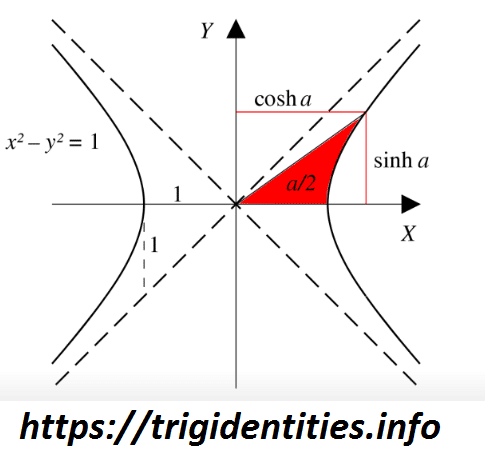# Hyperbolic Trig Identities

Hyperbolic Trig Identities is like trigonometric identities yet may contrast to it in specific terms. The fundamental hyperbolic functions are hyperbola sin and hyperbola cosine from which the other trigonometric functions are inferred. You can easily explore many other Trig Identities on this website.

So here we have given a Hyperbola diagram along these lines giving you thought regarding the places of sine, cosine, and so on.## Various Essential Hyperbolic Trig Identities

### Introduction

• x and y are independent variables.
• e is the base of the natural logarithm.
• d is the differential operator.
• int is the integral symbol.
• C is the constant of integration.

## Hyperbolic Trig Identities

sinh x = (ex – ex)/2 Equation 1
cosh x = (ex + ex)/2 Equation 2
sech x = 1/cosh x Equation 3
csch x = 1/sinh x Equation 4
tanh x = sinh x/cosh x Equation 5
coth x = 1/tanh x Equation 6
cosh2 x – sinh2 x = 1 Equation 7
tanh2 x + sech2 x = 1 Equation 8
coth2 x – csch2 x = 1 Equation 9
sinh (x + y) = sinh x cosh y + cosh x sinh y Equation 10
sinh (x – y) = sinh x cosh y – cosh x sinh y Equation 11
cosh (x + y) = cosh x cosh y + sinh x sinh y Equation 12
cosh (x – y) = cosh x cosh y – sinh x sinh y Equation 13
sinh2 x = [-1 + cosh (2 x)]/2 Equation 14
sinh (2 x) = 2 sinh x cosh x Equation 15
cosh2 x = [1 + cosh (2 x)]/2 Equation 16
cosh (2 x) = cosh2 x + sinh2 x Equation 17
arcsinh x = ln [x + (x2 + 1)1/2] Equation 18
arccosh x = ln [x + (x2 – 1)1/2] Equation 19
arctanh x = (1/2) ln [(1 + x)/(1 – x)] Equation 20
arccoth x = (1/2) ln [(x + 1)/(x – 1)] Equation 21
arcsech x = ln [[1 + (1 – x2)1/2]/x] Equation 22
arccsch x = ln [[1 + (1 + x2)1/2]/|x|] Equation 23
tanh (2 x) = 2 tanh x/(1 + tanh2 x) Equation 24
coth x – tanh x = 2 csch (2 x) Equation 25
coth x + tanh x = 2 coth (2 x) Equation 26
(d/dx) sinh x = cosh x Equation 27
(d/dx) cosh x = sinh x Equation 28
(d/dx) tanh x = sech2 x Equation 29
(d/dx) coth x = -csch2 x Equation 30
(d/dx) sech x = -sech x tanh x Equation 31
(d/dx) csch x = -csch x coth x Equation 32
(d/dx) arcsinh x = 1/(x2 + 1)1/2 Equation 33
(d/dx) arccosh x = 1/(x2 – 1)1/2 Equation 34
(d/dx) arctanh x = 1/(1 – x2) Equation 35
(d/dx) arccoth x = 1/(1 – x2) Equation 36
(d/dx) arcsech x = -1/[x (1 – x2)1/2] Equation 37
(d/dx) arccsch x = -1/[|x| (1 + x2)1/2] Equation 38
Integrals.
int cosh x dx = sinh x + C Equation 39
int sinh x dx = cosh x + C Equation 40
int sech2 x dx = tanh x + C Equation 41
int csch2 x dx = -coth x + C Equation 42
int sech x tanh x dx = -sech x + C Equation 43
int csch x coth x dx = -csch x + C Equation 44
int dx/(x2 + 1)1/2 = arcsinh x + C Equation 45
int dx/(x2 – 1)1/2 = arccosh x + C Equation 46
int dx/(1 – x2) = arctanh x + C Equation 47
int dx/(1 – x2) = arccoth x + C Equation 48
int dx/[x (1 – x2)1/2] = -arcsech x + C Equation 49
int dx/[|x| (1 + x2)1/2] = -arccsch x + C Equation 50